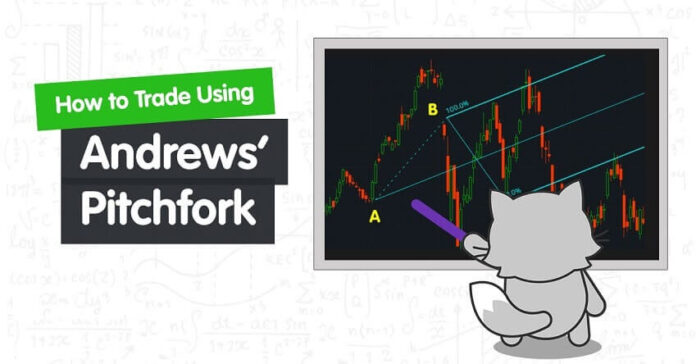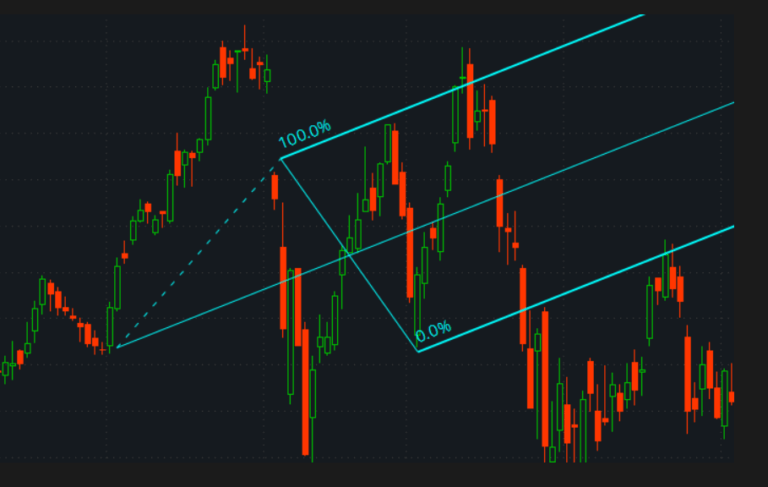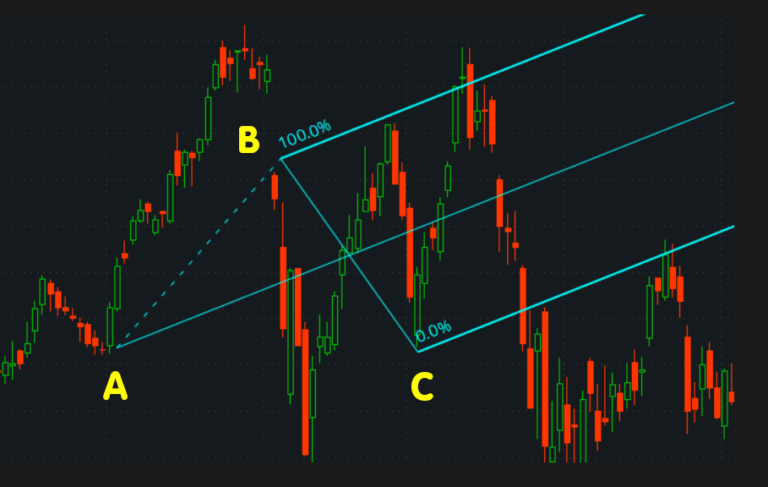# Andrews’ Pitchfork

0
36You are here:

Andrews’ Pitchfork is a channel-based analysis technique developed by Dr. Alan Andrews.

The channel drawing technique uses three parallel trend lines to show areas of support and resistance. The three parallel lines are created from three consecutive major peaks or troughs.

The trend lines automatically generated by plotting three points on a chart, each marking an important pivot point.It is suggested that one of the following schemes be used:

• In an uptrend, trough-peak-trough.
• In a downtrend, peak-trough-peak.

The first two points define the trendline, the last two points define the channel.

After the last two points are connected, the channel is completed, and the median line is added, the plot indeed resembles a fork.

The main rule of using Andrews’ Pitchfork is the same as for the channel:

The handle (median line) shows the strength of the trend:

• In a strong uptrend, the price tends to be above the median line.
• In a strong downtrend, the price tends to be above the median line.

Andrews’ Pitchfork can be transformed into Schiff and Modified Schiff Pitchfork.

• Schiff Pitchfork moves the start point of the handle line halfway to the base of the channel.
• Modified Schiff Pitchfork adjusts the handle start point by the distance equal to half a difference between price values of base points of the channel.

#### How to Draw?

Here is an example of an Andrews’ Pitchfork during an uptrend.1. First find the most recent low, which is Point A. This is where the middle tine or median line will begin.
2. Then find the highest move made from Point A. which is Point B.
3. The next point is found by looking for the lowest retracement move from Point B, which is Point C.

Point A rallies to Point B, then retraces to Point C, then resumes its uptrend.

Notice how when the uptrend reverses, the lower trend line that used to act as a support level has now become a resistance level.

The pitchfork shows continuous points of support and resistance.

Andrews’ Pitchfork tool allows traders to trade channels when the market is trending.

Generally, one would sell when the price rises to upper tine, which is line B, and take profit when the price reaches the middle tine, which is line A

One would also buy if the price falls to the lower tine, which is line C, and take profit when it rises back to the middle tine, line A.